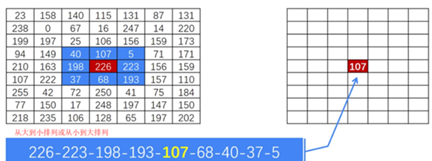﻿ Python OpenCV图像处理之图像滤波特效详解_python_脚本之家
python# Python OpenCV图像处理之图像滤波特效详解

## 1 分类

• 邻域滤波(Spatial Domain Filter)，其本质是数字窗口上的数学运算。一般用于图像平滑、图像锐化、特征提取(如纹理测量、边缘检测)等，邻域滤波使用邻域算子——利用给定像素周围像素值以决定此像素最终输出的一种算子
• 频域滤波(Frequency Domain Filter)，其本质是对像素频率的修改。一般用于降噪、重采样、图像压缩等。

• 低通滤波。滤除原图像的高频成分，即模糊图像边缘与细节。
• 高通滤波。滤除原图像的低频成分，即图像锐化。

```import cv2,skimage
import numpy as np

# 原图
cv2.imshow("src image", srcImg)

# 给图像增加高斯噪声
noiseImg = skimage.util.random_noise(srcImg, mode='gaussian')
cv2.imshow("image with noise", noiseImg)```1. gaussian：高斯加性噪声
2. localvar：高斯加性噪声，每点具有特定局部方差
3. poisson： 泊松分布噪声
4. salt：盐噪声，随机用1替换像素
5. pepper：胡椒噪声，随机用0或-1替换像素
6. s&p：椒盐噪声，呈现出黑白杂点

## 2 邻域滤波

### 2.1 线性滤波

2.1.1 方框滤波```# 方框滤波
boxImg = cv2.boxFilter(noiseImg, ddepth = -1, ksize = (2, 2), normalize = False)
cv2.imshow("box Image", boxImg)
# 均值滤波
blurImg = cv2.blur(noiseImg, (6, 5))
cv2.imshow("blur image", blurImg)
```2.1.2 高斯滤波• 核大小，其决定了图像的平滑范围。理论上，高斯核函数应该无穷大，以达到最佳的平滑效果，但过大的卷积核会导致运算效率骤降。根据高斯函数3σ规则，可以取高斯核大小为(6σ+1)×(6σ+1)；
• 离散程度σ，其决定了对高频成分的抑制程度。σ越大，像素加权半径越大，平滑程度越强。```# 高斯滤波
gaussImg = cv2.GaussianBlur(noiseImg, (5, 5), 0)
cv2.namedWindow("gaussain image")
cv2.imshow("gaussain image", gaussImg)
```### 2.2 非线性滤波```# 原图
cv2.imshow("src image", srcImg)

# 给图像增加椒盐噪声
noiseImg = skimage.util.random_noise(srcImg, mode='s&p')
cv2.imshow("image with noise", noiseImg)

medImg = cv2.medianBlur(np.uint8(noiseImg * 255), 3)
cv2.namedWindow("median image")
cv2.imshow("median image", medImg)
```## 3 频域滤波```# 傅里叶变换
dft = cv2.dft(np.float32(grayImg), flags = cv2.DFT_COMPLEX_OUTPUT)
# 将图像中的低频部分移动到图像的中心
dftShift = np.fft.fftshift(dft)
# 计算幅频特性
magnitude = 20 * np.log(cv2.magnitude(dftShift[:, :, 0], dftShift[:, :, 1]))
plt.subplot(121), plt.imshow(grayImg, cmap = 'gray')
plt.title('原图'), plt.xticks([]), plt.yticks([])
plt.subplot(122), plt.imshow(magnitude, cmap = 'gray')
plt.title('频谱图'), plt.xticks([]), plt.yticks([])
plt.show()
```

### 3.1 低通滤波

```# 定义滤波掩码
crow, ccol = int(img.shape / 2), int(img.shape / 2) # 求得图像的中心点位置
# 低通
if ftype == 'low':
mask = np.zeros((img.shape, img.shape, 2), np.uint8)
# 高通
if ftype == 'high':
mask = np.ones((img.shape, img.shape, 2), np.uint8)

lowImg = dftShift * mask(grayImg, 'low')
lowImg = np.fft.ifftshift(lowImg)
lowImg = cv2.idft(lowImg)
lowImg = cv2.magnitude(lowImg[:, :, 0], lowImg[:, :, 1])
```### 3.2 高通滤波

```# 定义滤波掩码
crow, ccol = int(img.shape / 2), int(img.shape / 2) # 求得图像的中心点位置
# 低通
if ftype == 'low':
mask = np.zeros((img.shape, img.shape, 2), np.uint8)
# 高通
if ftype == 'high':
mask = np.ones((img.shape, img.shape, 2), np.uint8)